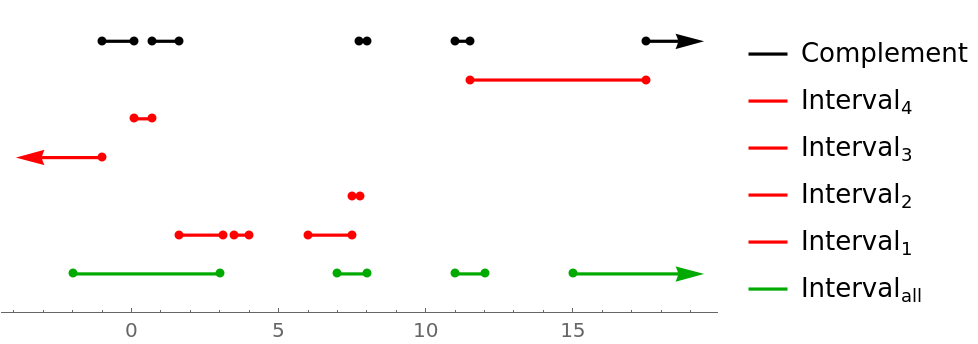#Function Repository Resource:

# IntervalComplement

Calculate the complement of intervals

Contributed by: Sander Huisman
 ResourceFunction["IntervalComplement"][intervalall,interval1,interval2,…] gives the interval representing all the points of intervalall that are not in any of the intervali.

## Details and Options

ResourceFunction["IntervalComplement"] only works on Interval objects with bounds that are real and not symbolic.
ResourceFunction["IntervalComplement"] supports the intervals to have -∞ and +∞ bounds.

## Examples

### Basic Examples

Calculate the complement of two intervals:

 In:=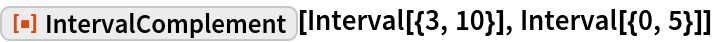Out=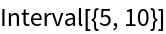Intervals can be disjoint:

 In:=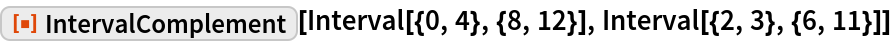Out=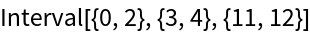### Scope

Subtract two Interval objects from another interval object:

 In:=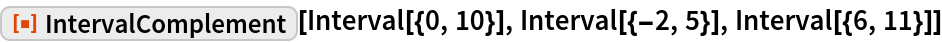Out=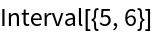Subtracting an interval might create a disjoint interval:

 In:=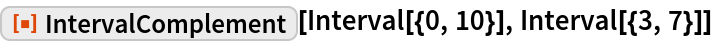Out=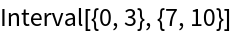Unbounded intervals can be used:

 In:=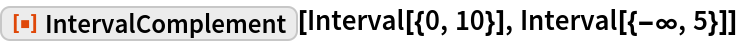Out=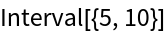In:=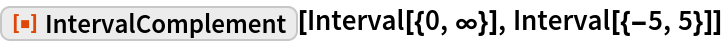Out=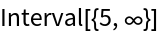In:=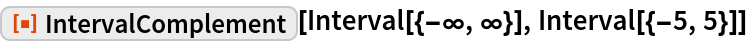Out=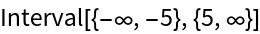If there is full overlap, an empty interval is returned:

 In:=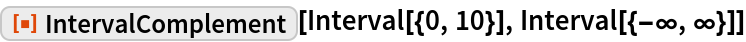Out=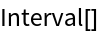An empty interval stays empty:

 In:=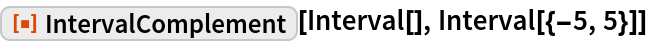Out=Complementing with an empty interval has no effect:

 In:=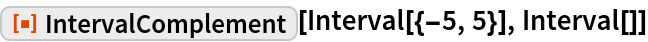Out=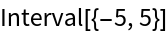### Applications

Calculate the absolute complement of the interval [-5,5] by taking the relative complement with the full interval:

 In:=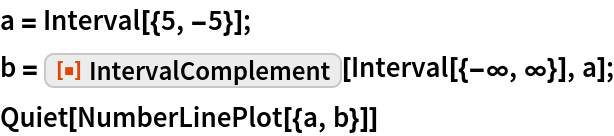Out=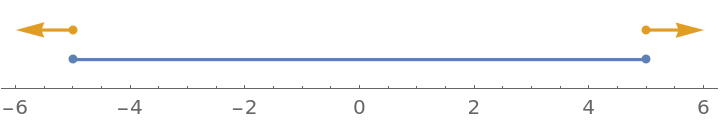### Properties and Relations

Compare the complement with the union and the intersection of two intervals:

 In:=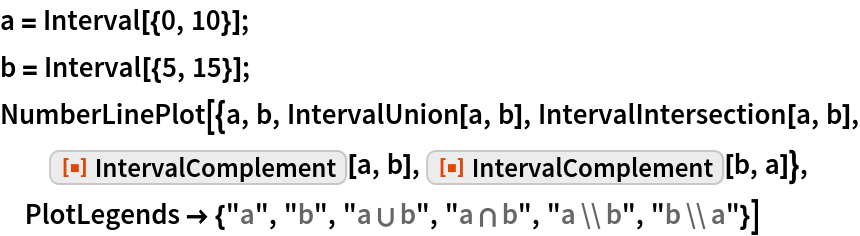Out=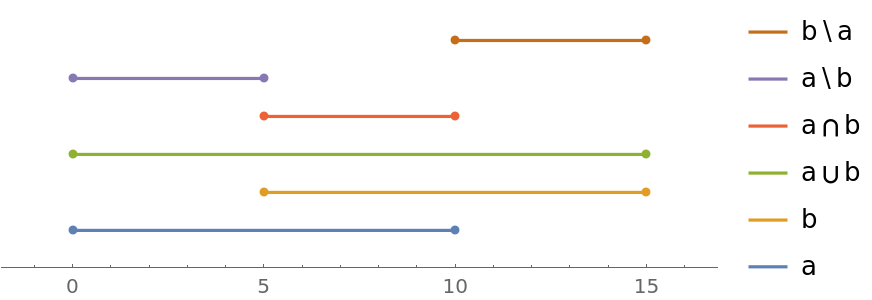Compare to regular sets:

 In:=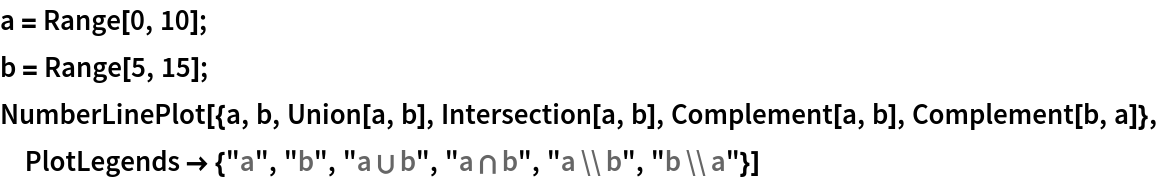Out=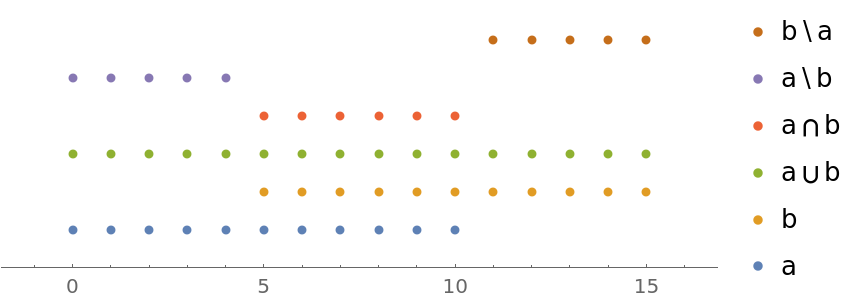### Possible Issues

Intervals need to be numeric. If the input is symbolic, it will stay unevaluated:

 In:=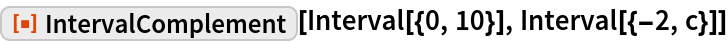Out=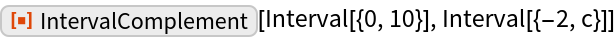### Neat Examples

Visualize the subtraction of multiple intervals (red) from a base interval (green):

 In:=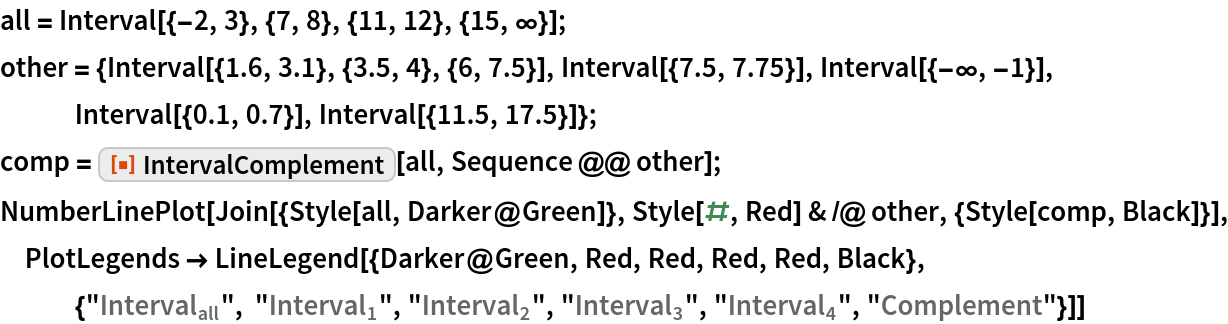Out=